Cooling Tower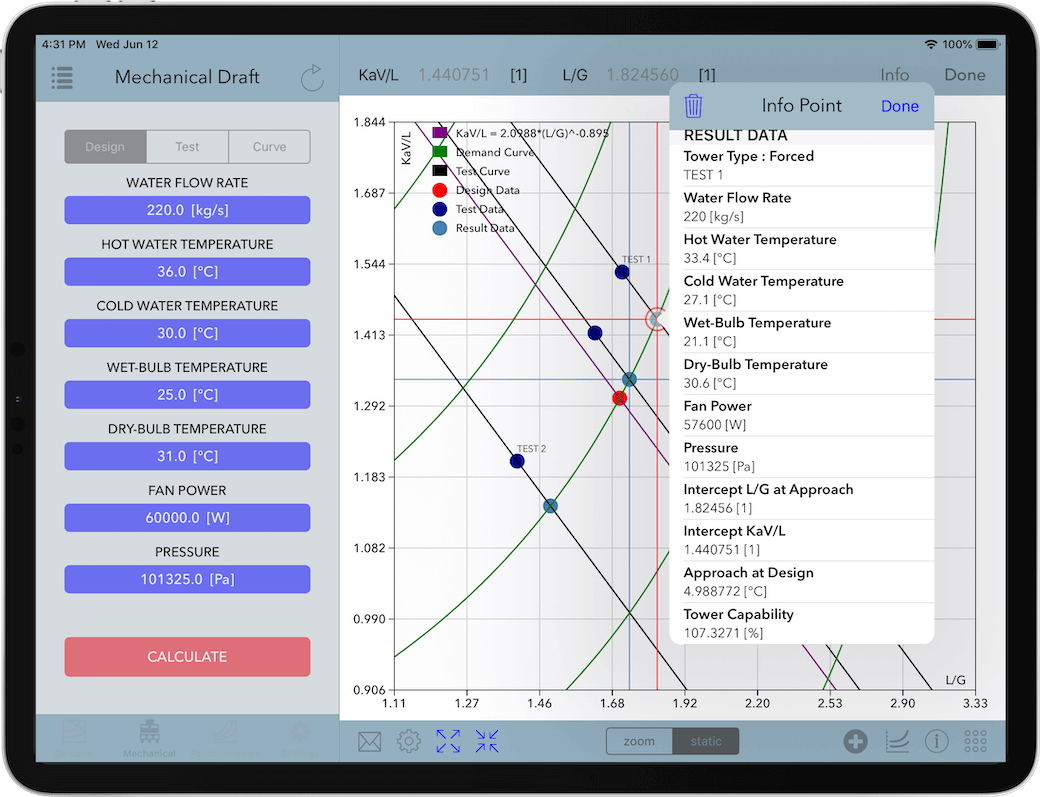#### REQUIREMENTS

• Requires iOS 12.1 or later. Compatible with iPad.

#### FEATURES

• Numerical calculation and graphical display of cooling capability and thermal performance of mechanical draft cooling towers based on the Acceptance Test Code CTI ATC-105 using the characteristic curve method (counterflow and crossflow, induced and forced draft).
• Calculation and graphical display of demand curves and approach points.
• Calculation of Merkel number using the Chebyshev numerical method.
• A psychrometrics calculator based on the latest mathematical models to numerically evaluate the properties of humid air, water, steam, ice and psychrometrics.
• Validates each calculation informing the user of the range of values to be entered in case of an invalid input.
• It manages and converts results and inputs between the SI (metric) and I-P (english) System of Units.
• Calculation projects and results can be saved to a database for later retrieval.
• Numerical results can be sent by email along with a comma-separated value (CSV) file. Calculation Plots can be sent as high-resolution pdf files.

# Computational suite to analyze and determine the cooling capability and performance of mechanical draft cooling towers (counterflow and crossflow, induced and forced draft).

• Mechanical Draft Cooling Towers.
• Demand Curves.
• Merkel Number.
• Psychrometrics Calculator.

### Mechanical Draft

#### Numerical calculation and graphical display of performance and thermal capability based on the Acceptance Test Code CTI ATC-105 using the characteristic curve method for mechanical draft cooling towers (counterflow and crossflow, induced and forced draft).

• Evaluation of the performance of mechanical draft cooling towers based on the Acceptance Test Code CTI ATC-105 from test data points using the characteristic curve method.
• Calculation of tower cooling capability for each data test added to a project.
• Calculation and graphical display of demand curves following the integration of Merkel´s equation.
• Complete validation of input variables, informing the user of the correct range of variables for a valid test data calculation.
• Creation of projects in a database that describe a particular set of design and test input data together with the calculated demand curves, test data and results for later retrieval or recalculation.
• Generation of high-quality pdf files of demand curve plots and the corresponding design, test and result points for a particular project.
• Generation of comma-separated values (CSV) files from numerical results of data tests.
• Supports input variables and calculation results in SI (metric) and I-P (english) system of units.

### Demand Curves

#### Numerical calculation and graphical display of demand curves and approach data points.

• Calculation and graphical display of demand curves following the integration of Merkel´s equation.
• Calculation and graphical display of approach data points.
• Complete validation of input variables.
• Creation of projects in a database that describe a particular set of input variables together with the calculated demand curves and approach data points for later retrieval or recalculation.
• Generation of high-quality pdf files of demand curve plots.
• Generation of comma-separated values (CSV) files from approach data point calculations results.
• Supports input variables and calculation results in SI (metric) and I-P (english) system of units.

### Merkel Number

#### Numerical calculation of Merkel number using the Chebyshev numerical method.

• Calculation of Merkel number using the Chebyshev numerical method.
• Complete validation of input variables, informing of the correct range of variables for a valid calculation.
• Supports input variables and calculation results in SI (metric) and I-P (english) system of units.

### Psychrometrics Calculator

#### A psychrometrics calculator based on the latest mathematical models to numerically evaluate the properties of humid air, water, steam and ice.

• Calculation of 41 properties of humid air, water, steam, ice and psychrometrics.
• It allows for 17 combinations of two thermodynamic properties to be entered as input parameters.
• Complete validation of input thermodynamic properties, it calculates and provides the user with information about the appropriate input values in the valid range of computations.
• Supports input variables and calculation results in SI (metric) and I-P (english) system of units.

### Merkel Number

#### Introduction

An evaporative cooling tower is a device that is used to remove waste heat from the water used in an industrial process equipment or a machinery by rejecting that waste heat into the environment. When water is mixed with air in a cooling tower configuration, a heat transfer process takes places that involves a latent heat transfer due to the vaporization of a small amount of water and a sensible heat transfer reflecting the difference in temperatures of water and air.
Based on the theory developed by Merkel, the heat transfer process that occurs in a cooling tower by considering the enthalpy potential difference as the driving force is described by the Merkel equation:

$\frac{{KaV}}{L} = \int_{{T_2}}^{{T_1}} {\frac{{{c_{pw}}d{T_w}}}{{h' - h}}}$
(1)
where:
 $\frac{{KaV}}{L}$ $=$ Tower characteristic $T{}_1$ $=$ Hot water temperature (inlet) $T{}_2$ $=$ Cold water temperature (outlet) $h'$ $=$ Enthalpy of saturated air at water temperature $h$ $=$ Enthalpy of main airstream ${c_{pw}}$ $=$ Specific heat capacity of water $d{T_w}$ $=$ Temperature differential of water

The Merkel Number application solves the equation (1) numerically using the four-point Chebyshev numerical method employing the following models for the calculation of water and air properties:

Properties of Water and Steam

• Formulations from the IAPWS (International Association for the Properties of Water and Steam) IAPWS-IF97 Industrial formulation (Revision 2007) and related models.

Properties of Humid Air

• Thermodynamic and psychrometric property algorithms from the ASHRAE Research Project 1485.
• Scientific Formulation IAPWS-95, IAPWS Formulation 2008 and IAPWS Formulation 2006. Properties of dry air are from the NIST Reference equation of Lemmon et al.

#### Graphical User Interface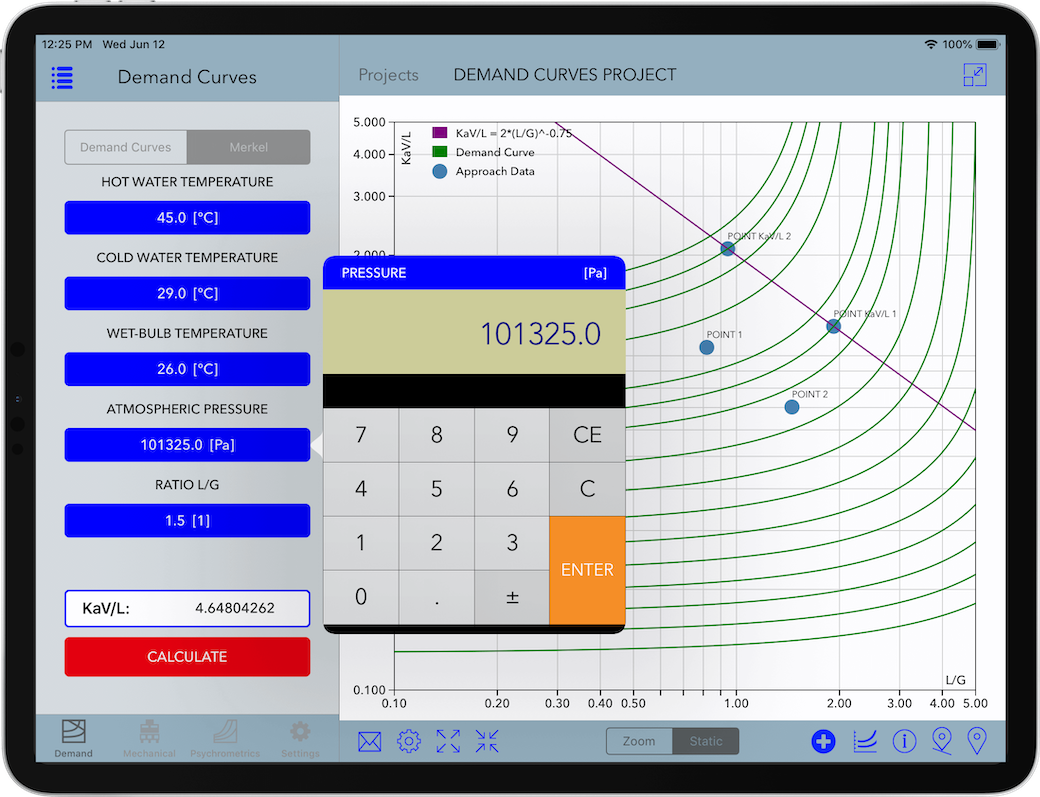Figure 1. Merkel Number calculation in Cooling Tower.

#### Range of Input Variables

Property Range in SI Units SI Units
HOT WATER TEMPERATURE 1.0 ≤ T ≤ 90.0 °C
COLD WATER TEMPERATURE 1.0 ≤ T ≤ 90.0 °C
WET-BULB TEMPERATURE 1.0 ≤ T ≤ 90.0 °C
PRESSURE 60000 ≤ P ≤ 110000 Pa
RATIO L/G 0.01 ≤ L/G ≤ 5.0 1
Table 1. Full ranges of input variables for calculation of Merkel Number in SI Units.

Property Range in I-P Units I-P Units
HOT WATER TEMPERATURE 33.8 ≤ T ≤ 194.0 °F
COLD WATER TEMPERATURE 33.8 ≤ T ≤ 194.0 °F
WET-BULB TEMPERATURE 33.8 ≤ T ≤ 194.0 °F
PRESSURE 8.70226426 ≤ P ≤ 15.95415115 psia
RATIO L/G 0.01 ≤ L/G ≤ 5.0 1
Table 2. Full ranges of input variables for calculation of Merkel Number in I-P Units.

### Demand Curves

#### Introduction

Demand Curves allows to calculate and plot in a log-log graph isolines resulting from the integration of equation (1) using as a parameter an approach value. It also calculates the approach given a pair of values determined by KaV/L and L/G, both in the SI and I-P system of units. The definition of the input variables for calculation of the demand curves is given in Table X.

For a specific tower, there is a characteristic curve in the form of a plot of tower characteristic, $KaV/L$, versus  water to air flow ratio, $L/G$. This plot is described with an equation of the following form:

$\frac{{KaV}}{L} = c{\left( {\frac{L}{G}} \right)^{ - n}}$
(2)

Where $L$ = water flow rate; $G$ = airflow rate; $c$ = constant defined for a particular packing design, or the intercept of the characteristic curve at $L/G$ = 1; $n$ = exponent related to packing design determined from test data.

#### Graphical User Interface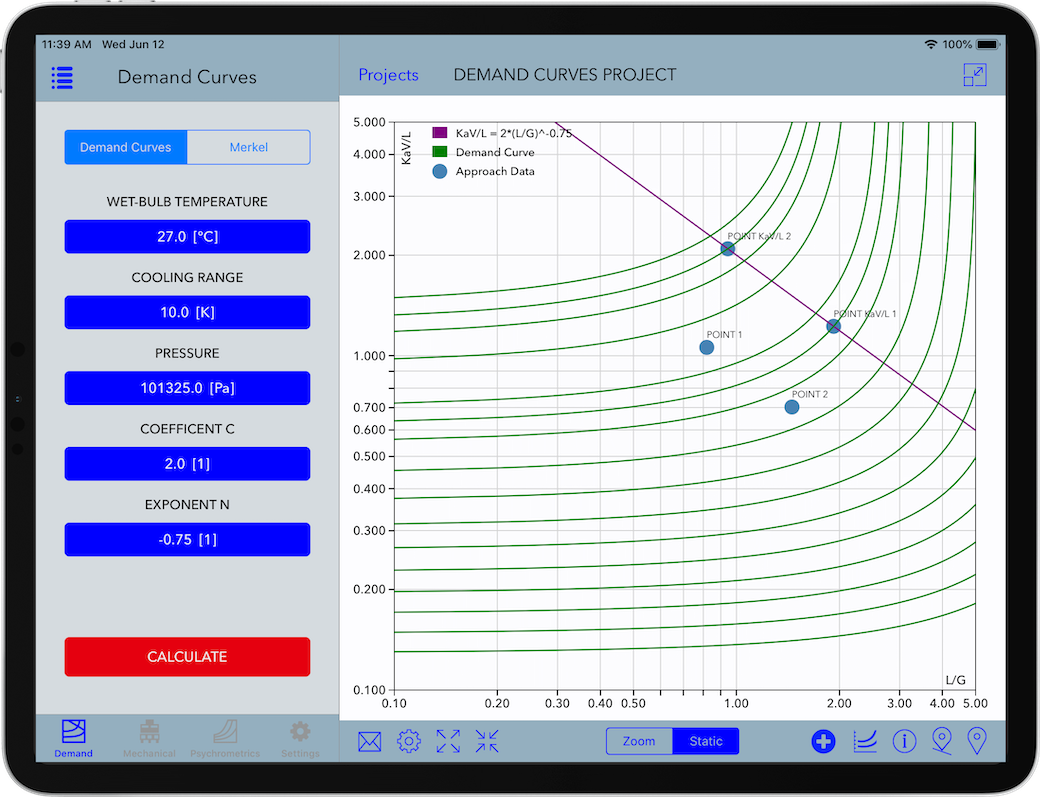Figure 2. Graphical User Interface for Demand Curves.

Input Variable Definition
WET-BULB TEMPERATURE Temperature of air wet-bulb entering the cooling tower
COOLING RANGE Difference between hot water temperature and cold water temperature
PRESSURE Total pressure referred to atmospheric
COEFFICIENT C Constant defined for a particular packing design
EXPONENT N Exponent defined for a particular packing design
Table 3. Definition of input variables in Demand Curves.

#### Range of Input Variables

Property Range in SI Units SI Units
WET-BULB TEMPERATURE 1.0 ≤ T ≤ 90.0 °C
COOLING RANGE 0.1 ≤ T ≤ 90.0 °C
PRESSURE 60000 ≤ P ≤ 110000 Pa
COEFFICIENT C 1.0 ≤ C ≤ 3.0 1
EXPONENT N -2.0 ≤ N ≤ -0.1 1
KaV/L 0.1 ≤ KaV/L ≤ 5.0 1
L/G 0.1 ≤ L/G ≤ 5.0 1
Approach 1.0 ≤ T ≤ 60.0 °C
Table 4. Full ranges of input variables in Demand Curves (SI Units).

Property Range in SI Units SI Units
WET-BULB TEMPERATURE 33.8 ≤ T ≤ 194.0 °F
COOLING RANGE 0.1 ≤ T ≤ 162.0 °F
PRESSURE 8.70226426 ≤ P ≤ 15.95415115 psia
COEFFICIENT C 1.0 ≤ C ≤ 3.0 1
EXPONENT N -2.0 ≤ N ≤ -0.1 1
KaV/L 0.1 ≤ KaV/L ≤ 5.0 1
L/G 0.1 ≤ L/G ≤ 5.0 1
Approach 1.0 ≤ T ≤ 140.0 °F
Table 5. Full ranges of input variables in Demand Curves (I-P Units).

#### Calculation Results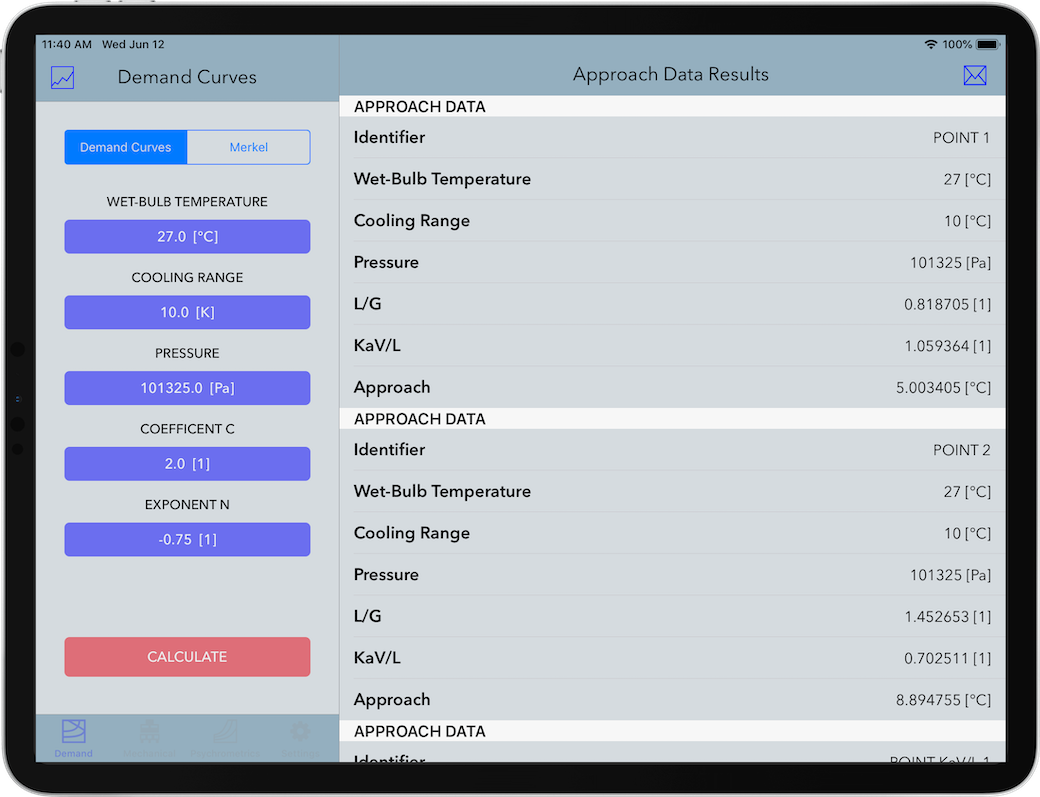Figure 3. Numerical Results in a Demand Curves calculation project.

#### Introduction

Mechanical Draft allows the evaluation of the performance capability of mechanical draft cooling towers based on the Acceptance Test Code CTI ATC-105 from test data points using the characteristic curve method.

By determining design and test conditions for a particular mechanical draft cooling tower, the manufacturer´s characteristic curve parameters and a design liquid to gas ratio (L/G), Mechanical Draft calculates the cooling capability at design conditions, plotting in a log-log graph each of the input data conditions as well as the resulting intercept results (denoted as a result data) given as a pair of values determined by KaV/L and L/G in the SI and I-P system of units.

Mechanical Draft solves the energy equation by integrating the Merkel equation numerically using the four-point Chebyshev numerical method employing the following models for the calculation of water and air properties:

Properties of Water and Steam

• Formulations from the IAPWS (International Association for the Properties of Water and Steam) IAPWS-IF97 Industrial formulation (Revision 2007) and related models.

Properties of Humid Air

• Thermodynamic and psychrometric property algorithms from the ASHRAE Research Project 1485.
• Scientific Formulation IAPWS-95, IAPWS Formulation 2008 and IAPWS Formulation 2006. Properties of dry air are from the NIST Reference equation of Lemmon et al.

#### Graphical User Interface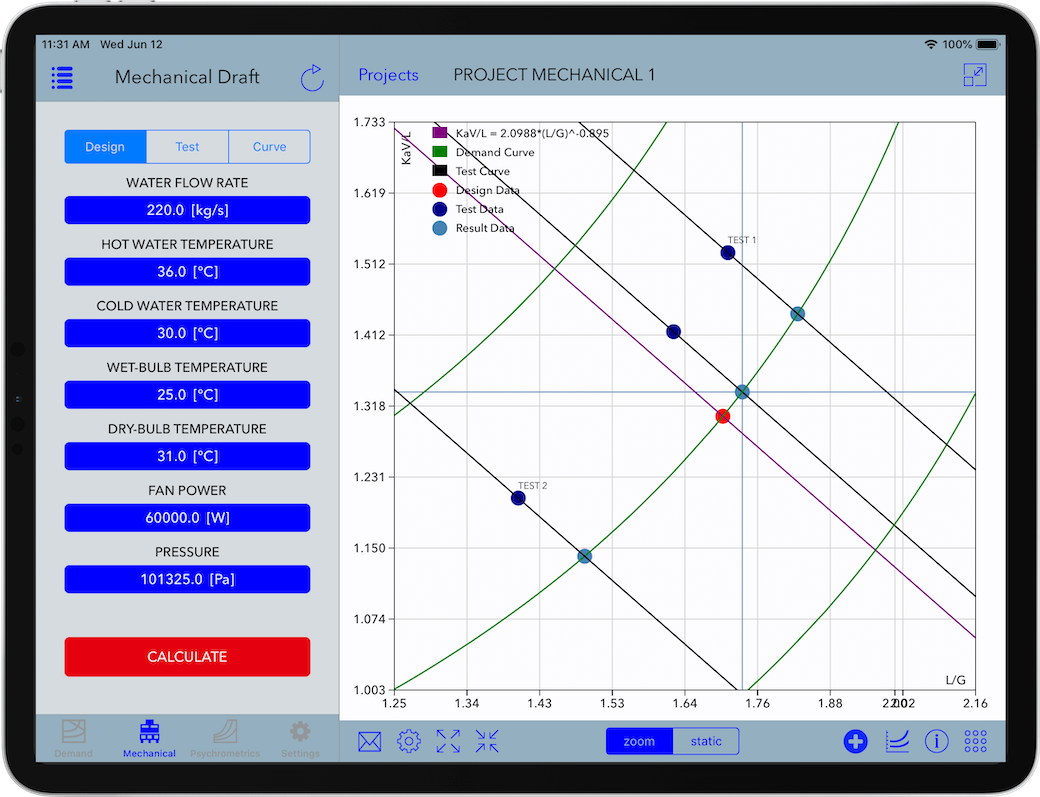Figure 1. Graphical User Interface in Mechanical Draft.

Input Variable Definition
WATER FLOW RATE Quantity of hot water flowing into the tower.
HOT WATER TEMPERATURE Temperature of inlet water.
COLD WATER TEMPERATURE Average temperature of the cold water basin discharge (outlet).
WET-BULB TEMPERATURE Temperature of air wet-bulb entering the cooling tower.
DRY-BULB TEMPERATURE Temperature of air dry-bulb entering the cooling tower.
FAN POWER Power input to the fan drive assembly, excluding power losses in the driver.
PRESSURE Total pressure referred to atmospheric.
COEFFICIENT C Constant defined for a particular packing design.
EXPONENT N Exponent defined for a particular packing design.
DESIGN L/G RATIO C Ratio of water flow rate to airflow rate at design.
TOWER TYPE $Forced$ draft: fan located near the bottom, forcing the air from bottom to top.
$Induced$ draft: fan located at the top inducing suction from the tower and discharging it into the atmosphere.
Table 1. Definition of input variables in Mechanical Draft.

#### Range of Input Variables

Property Range in SI Units SI Units
WATER FLOW RATE 0.5 ≤ Flow ≤ 100000.0 kg/s
HOT WATER TEMPERATURE 1.0 ≤ T ≤ 90.0 °C
COLD WATER TEMPERATURE 1.0 ≤ T ≤ 90.0 °C
WET-BULB TEMPERATURE 1.0 ≤ T ≤ 90.0 °C
DRY-BULB TEMPERATURE 1.0 ≤ T ≤ 90.0 °C
FAN POWER 1.0 ≤ Fan ≤ 1.0E6 W
PRESSURE 60000 ≤ P ≤ 110000 Pa
COEFFICIENT C 1.0 ≤ C ≤ 3.0 1
EXPONENT N -2.0 ≤ N ≤ -0.1 1
DESIGN L/G RATIO 0.1 ≤ L/G ≤ 5.0 1
KaV/L 0.1 ≤ KaV/L ≤ 5.0 1
L/G 0.1 ≤ L/G ≤ 5.0 1
APPROACH 1.0 ≤ T ≤ 60.0 °C
Table 2. Full ranges of input variables in Mechanical Draft (SI Units).

Property Range in I-P Units I-P Units
WATER FLOW RATE 7.92 ≤ Flow ≤ 1585032.22 gpm
HOT WATER TEMPERATURE 33.8 ≤ T ≤ 194.0 °F
COLD WATER TEMPERATURE 33.8 ≤ T ≤ 194.0 °F
WET-BULB TEMPERATURE 33.8 ≤ T ≤ 194.0 °F
DRY-BULB TEMPERATURE 33.8 ≤ T ≤ 194.0 °F
FAN POWER 0.001342 ≤ Fan ≤ 1341.022 bhp
PRESSURE 8.702264 ≤ P ≤ 15.954151 psia
COEFFICIENT C 1.0 ≤ C ≤ 3.0 1
EXPONENT N -2.0 ≤ N ≤ -0.1 1
DESIGN L/G RATIO 0.1 ≤ L/G ≤ 5.0 1
KaV/L 0.1 ≤ KaV/L ≤ 5.0 1
L/G 0.1 ≤ L/G ≤ 5.0 1
APPROACH 1.0 ≤ T ≤ 140.0 °F
Table 3. Full ranges of input variables in Mechanical Draft (I-P Units).

#### Calculation Results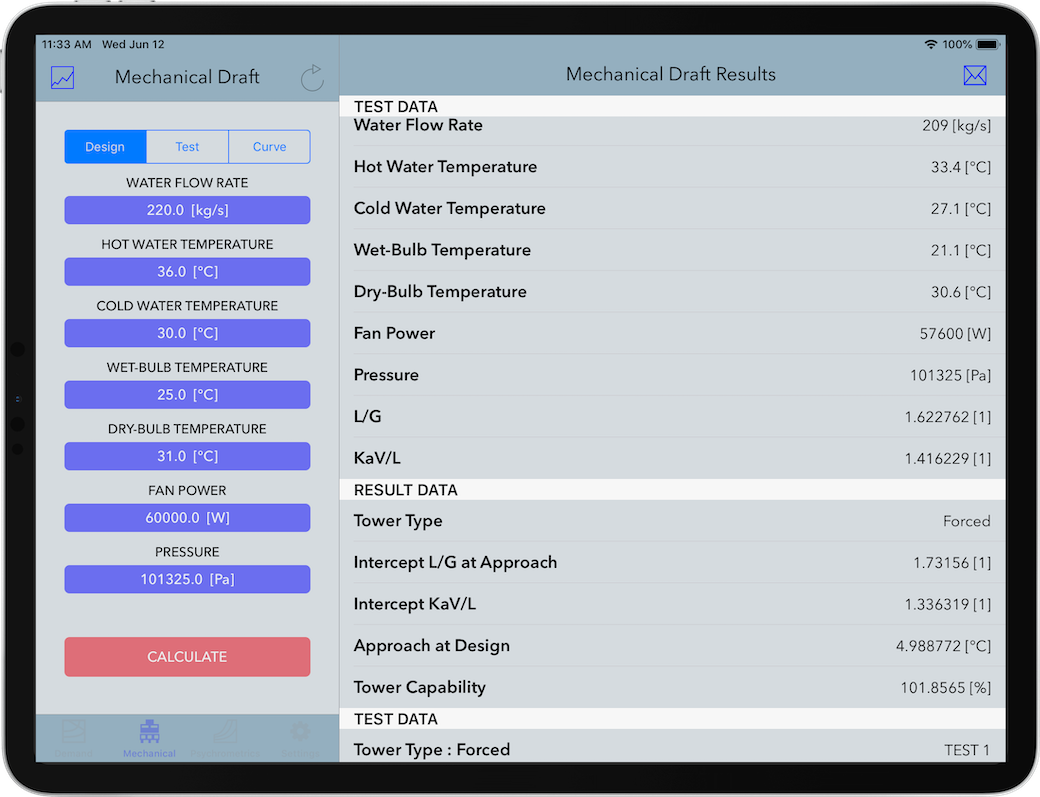Figure 2. Numerical Results in a Mechanical Draft calculation project.

Result Variable Definition
APPROACH Approach calculated at design data.
INTERCEPT L/G AT APPROACH Value of L/G ratio calculated at result point (L/G value of the intersection of the demand curve calculated on the approach at design and the curve parallel to the characteristic curve defined on the test point).
INTERCEPT KaV/L Value of KaV/L ratio calculated at result point (KaV/L value of the intersection of the demand curve calculated on the approach at design and the curve parallel to the characteristic curve defined on the test point).
TOWER CAPABILITY Cooling capability result calculated for a data test.
Table 4. Definition of result variables in Mechanical Draft.

#### Introduction

Psychrometrics Calculator allows the calculation of physical properties of humid air, water, steam, ice and psychrometrics commonly used in the design and operation of cooling towers.

• Calculation of 41 properties of humid air, water, steam, ice and psychrometrics.
• Allows for 17 combinations of two thermodynamic properties to be entered as input variables:
• Dry-bulb Temperature / Wet-bulb Temperature
• Dry-bulb Temperature / Dew Point Temperature
• Dry-bulb Temperature / Relative Humidity
• Dry-bulb Temperature / Humidity Ratio
• Dry-bulb Temperature / Specific Enthalpy
• Dry-bulb Temperature / Specific Volume
• Wet-bulb Temperature / Dew Point Temperature
• Wet-bulb Temperature / Relative Humidity
• Wet-bulb Temperature / Humidity Ratio
• Dew Point Temperature / Relative Humidity
• Dew Point Temperature / Specific Enthalpy
• Dew Point Temperature / Specific Volume
• Relative Humidity / Humidity Ratio
• Relative Humidity / Specific Enthalpy
• Relative Humidity / Specific Volume
• Humidity Ratio / Specific Enthalpy
• Humidity Ratio / Specific Volume
• Supports input parameters and calculation results in both SI (metric) and I-P (english) system of units.
• For each combination of input thermodynamic variables, it calculates and provides the user with information about the appropriate values in the valid range of computations.
• Calculation results can be saved to a to a database for later retrieval.
• Calculation results can be exported to excel/pdf file formats.

Calculation of the properties of humid air, water, steam, ice and psychrometrics are based on the precision provided by the mathematical formulations of the following thermodynamic and transport properties models:

Properties of Water and Steam

• Formulations from the IAPWS (International Association for the Properties of Water and Steam) IAPWS-IF97 Industrial formulation (Revision 2007) and related models.

Properties of Humid Air

• Thermodynamic and psychrometric property algorithms from the ASHRAE Research Project 1485.
• Scientific Formulation IAPWS-95, IAPWS Formulation 2008 and IAPWS Formulation 2006. Properties of dry air are from the NIST Reference equation of Lemmon et al.

#### Graphical User Interface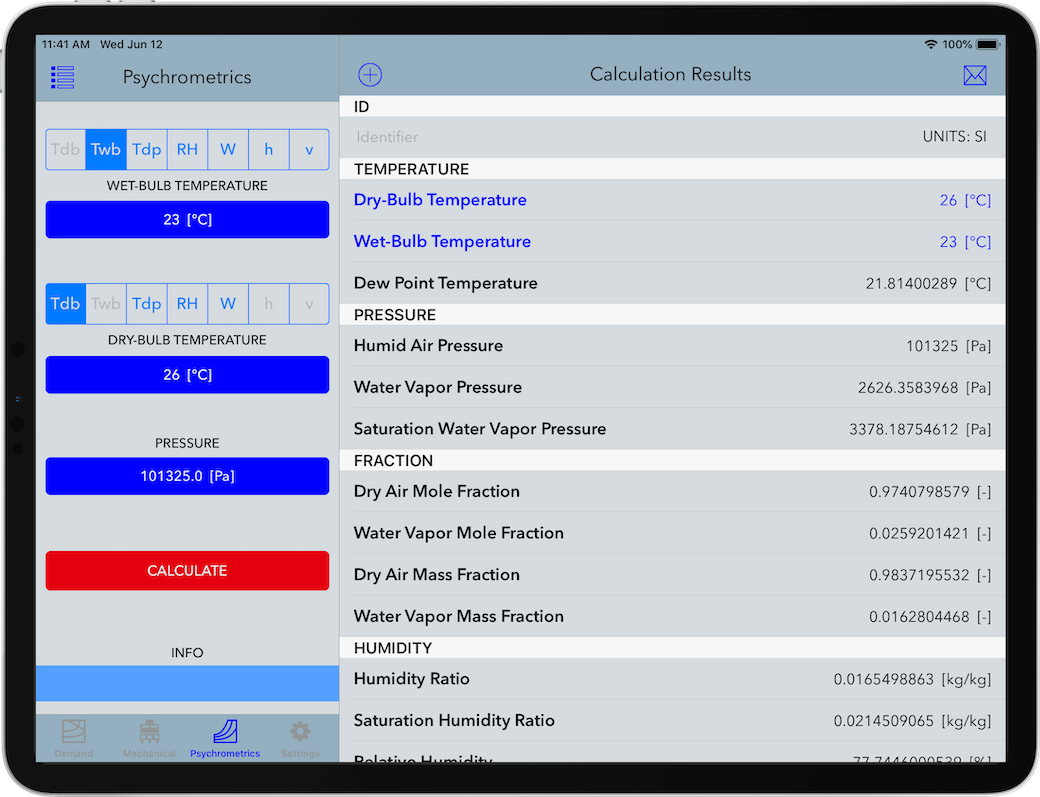Figure 1. Graphical User Interface in Psychrometrics Calculator.

#### Range of Input Variables

Property Range in SI Units SI Units
DRY-BULB TEMPERATURE -143.15 ≤ Tdb ≤ 350.0 °C
WET-BULB TEMPERATURE -143.15 ≤ Twb ≤ 350.0 °C
DEW POINT TEMPERATURE -143.15 ≤ Tdp ≤ 350.0 °C
RELATIVE HUMIDITY 0 .0 ≤ RH ≤ 100.0 [%]
HUMIDITY RATIO 0.0 ≤ W ≤ 10.0 kg/kg
SPECIFIC ENTHALPY -311.357 ≤ h ≤ 32135.848 kJ/kg
SPECIFIC VOLUME 1.469E-3 ≤ v ≤ 3.055E5 m3/kg
PRESSURE 10.0 ≤ P ≤ 10.0E6 Pa
Table 1. Full range of input variables in Psychrometrics Calculator (SI Units).

Property Range in I-P Units I-P Units
DRY-BULB TEMPERATURE -225.67 ≤ Tdb ≤ 662.0 °F
WET-BULB TEMPERATURE -225.67 ≤ Twb ≤ 662.0 °F
DEW POINT TEMPERATURE -225.67 ≤ Tdp ≤ 662.0 °F
RELATIVE HUMIDITY 0 .0 ≤ RH ≤ 100.0 [%]
HUMIDITY RATIO 0.0 ≤ W ≤ 10.0 lb/lb
SPECIFIC ENTHALPY -126.174 ≤ h ≤ 13823.61 Btu/lb
SPECIFIC VOLUME 2.353E-2 ≤ v ≤ 4.893E6 ft3/lb
PRESSURE 0.00145 ≤ P ≤ 1450.4 psia
Table 2. Full range of input variables in Psychrometrics Calculator (I-P Units).

#### Calculation Results

Property SI Units I-P Units
Dry-Bub Temperature °C °F
Wet-Bulb Temperature °C °F
Dew Point Temperature °C °F
Humid Air Pressure Pa, kPa, bar, mmHg psia, inHg, inH2O, atm
Water Vapor Partial Pressure Pa, kPa, bar, mmHg psia, inHg, inH2O, atm
Dry Air Partial Pressure Pa, kPa, bar, mmHg psia, inHg, inH2O, atm
Saturation Water Vapor Pressure Pa, kPa, bar, mmHg psia, inHg, inH2O, atm
Dry Air Mole Fraction [-] [-]
Water Mole Fraction [-] [-]
Dry Air Mass Fraction [-] [-]
Water Mass Fraction [-] [-]
Humidity Ratio kg(w)/kg(da), g(w)/kg(da) lb(w)/lb(da), gr(w)/lb(da)
Saturation Humidity Ratio kg(w)/kg(da), g(w)/kg(da) lb(w)/lb(da), gr(w)/lb(da)
Relative Humidity [%] [%]
Absolute Humidity kg(w)/m3 lb(w)/ft3
Parts per million by weight ppmw ppmw
Parts per million by volume ppmv ppmv
Enhancement Factor [-] [-]
Specific Volume of Dry Air m3/kg, cm3/g ft3/lb, in3/lb
Specific Volume of Humid Air m3/kg, cm3/g ft3/lb, in3/lb
Specific Volume of Saturated Water m3/kg, cm3/g ft3/lb, in3/lb
Specific Volume of Saturated Ice m3/kg, cm3/g ft3/lb, in3/lb
Specific Volume of Water Vapor m3/kg, cm3/g ft3/lb, in3/lb
Density of Dry Air kg/m3, g/cm3 lb/ft3, lb/in3
Density of Humid Air kg/m3, g/cm3 lb/ft3, lb/in3
Density of Saturated Water kg/m3, g/cm3 lb/ft3, lb/in3
Density of Saturated Ice kg/m3, g/cm3 lb/ft3, lb/in3
Density of Water Vapor kg/m3, g/cm3 lb/ft3, lb/in3
Specific Enthalpy of Dry Air J/kg, kJ/kg Btu/lb, ft lbf/lb
Specific Enthalpy of Humid Air J/kg, kJ/kg Btu/lb, ft lbf/lb
Specific Enthalpy of Saturated Water J/kg, kJ/kg Btu/lb, ft lbf/lb
Specific Enthalpy of Saturated Ice J/kg, kJ/kg Btu/lb, ft lbf/lb
Specific Enthalpy of Water Vapor J/kg, kJ/kg Btu/lb, ft lbf/lb
Specific Entropy of Dry Air J/(kg·K), kJ/(kg·K) Btu/(lb·°R), ft lbf/ (lb·°R)
Specific Entropy of Humid Air J/(kg·K), kJ/(kg·K) Btu/(lb·°R), ft lbf/ (lb·°R)
Specific Entropy of Saturated Water J/(kg·K), kJ/(kg·K) Btu/(lb·°R), ft lbf/ (lb·°R)
Specific Entropy of Saturated Ice J/(kg·K), kJ/(kg·K) Btu/(lb·°R), ft lbf/ (lb·°R)
Specific Entropy of Water Vapor J/(kg·K), kJ/(kg·K) Btu/(lb·°R), ft lbf/ (lb·°R)
Specific Internal Energy of Dry Air J/kg, kJ/kg Btu/lb, ft lbf/lb
Specific Internal Energy of Humid Air J/kg, kJ/kg Btu/lb, ft lbf/lb
Specific Isobaric Heat Capacity of Humid Air kJ/(kg·K) Btu/(lb·°R)
Compressibility of Humid Air [-] [-]
Table 3. Properties and their units calculated in Psychrometrics Calculator.

#### Notice

This website or its third-party tools use cookies, which are necessary to its functioning and required to achieve the purposes illustrated in the cookie policy. By closing this banner, scrolling this page, clicking a link or continuing to browse otherwise, you agree to the use of cookies. Please refer to our Cookie Policy, revised Privacy Policy and Terms and Conditions.